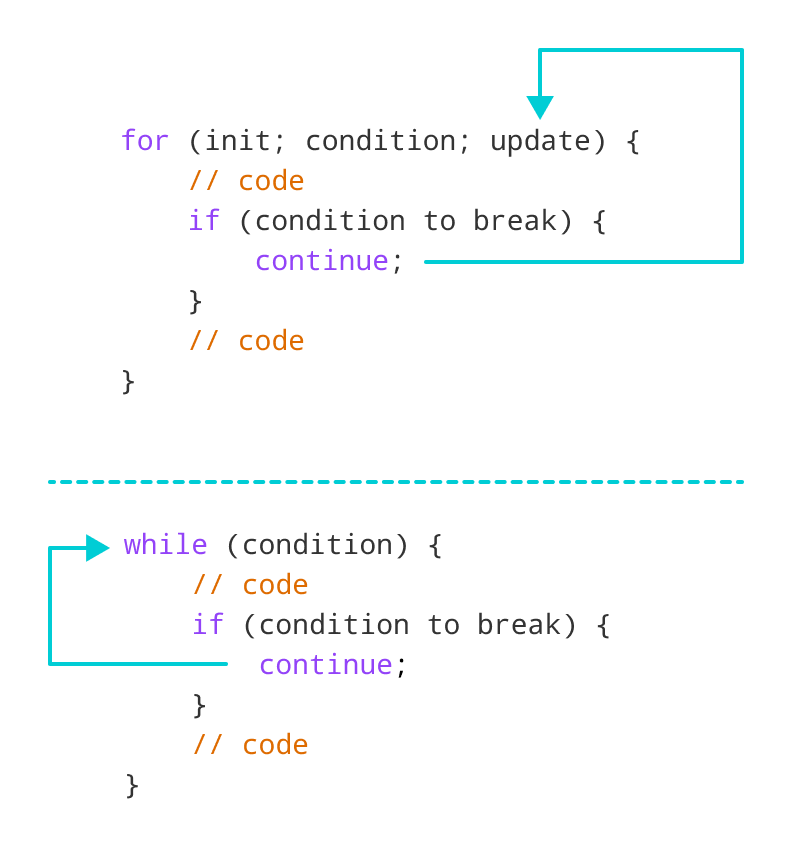# C++ continue Statement

In this tutorial, we will learn about the continue statement and its working with loops with the help of examples.

In computer programming, the `continue` statement is used to skip the current iteration of the loop and the control of the program goes to the next iteration.

The syntax of the `continue` statement is:

``continue;``

Before you learn about the continue statement, make sure you know about,

## Working of C++ continue StatementWorking of continue statement in C++

## Example 1: continue with for loop

In a `for` loop, `continue` skips the current iteration and the control flow jumps to the `update` expression.

``````// program to print the value of i

#include <iostream>
using namespace std;

int main() {
for (int i = 1; i <= 5; i++) {
// condition to continue
if (i == 3) {
continue;
}

cout << i << endl;
}

return 0;
}``````

Output

```1
2
4
5```

In the above program, we have used the the `for` loop to print the value of i in each iteration. Here, notice the code,

``````if (i == 3) {
continue;
}``````

This means

• When i is equal to `3`, the `continue` statement skips the current iteration and starts the next iteration
• Then, i becomes `4`, and the `condition` is evaluated again.
• Hence, `4` and `5` are printed in the next two iterations.

Note: The `continue` statement is almost always used with decision-making statements.

## Example 2: continue with while loop

In a `while` loop, `continue` skips the current iteration and control flow of the program jumps back to the `while` `condition`.

``````// program to calculate positive numbers till 50 only
// if the user enters a negative number,
// that number is skipped from the calculation

// negative number -> loop terminate
// numbers above 50 -> skip iteration

#include <iostream>
using namespace std;

int main() {
int sum = 0;
int number = 0;

while (number >= 0) {
// add all positive numbers
sum += number;

// take input from the user
cout << "Enter a number: ";
cin >> number;

// continue condition
if (number > 50) {
cout << "The number is greater than 50 and won't be calculated." << endl;
number = 0;  // the value of number is made 0 again
continue;
}
}

// display the sum
cout << "The sum is " << sum << endl;

return 0;
}``````

Output

```Enter a number: 12
Enter a number: 0
Enter a number: 2
Enter a number: 30
Enter a number: 50
Enter a number: 56
The number is greater than 50 and won't be calculated.
Enter a number: 5
Enter a number: -3
The sum is 99 ```

In the above program, the user enters a number. The `while` loop is used to print the total sum of positive numbers entered by the user, as long as the numbers entered are not greater than `50`.

Notice the use of the `continue` statement.

`````` if (number > 50){
continue;
}``````
• When the user enters a number greater than `50`, the `continue` statement skips the current iteration. Then the control flow of the program goes to the `condition` of `while` loop.
• When the user enters a number less than `0`, the loop terminates.

Note: The `continue` statement works in the same way for the `do...while` loops.

## continue with Nested loop

When `continue` is used with nested loops, it skips the current iteration of the inner loop. For example,

``````// using continue statement inside
// nested for loop

#include <iostream>
using namespace std;

int main() {
int number;
int sum = 0;

// nested for loops

// first loop
for (int i = 1; i <= 3; i++) {
// second loop
for (int j = 1; j <= 3; j++) {
if (j == 2) {
continue;
}
cout << "i = " << i << ", j = " << j << endl;
}
}

return 0;
}``````

Output

```i = 1, j = 1
i = 1, j = 3
i = 2, j = 1
i = 2, j = 3
i = 3, j = 1
i = 3, j = 3```

In the above program, when the `continue` statement executes, it skips the current iteration in the inner loop. And the control of the program moves to the update expression of the inner loop.

Hence, the value of j = 2 is never displayed in the output.

Note: The break statement terminates the loop entirely. However, the `continue` statement only skips the current iteration.